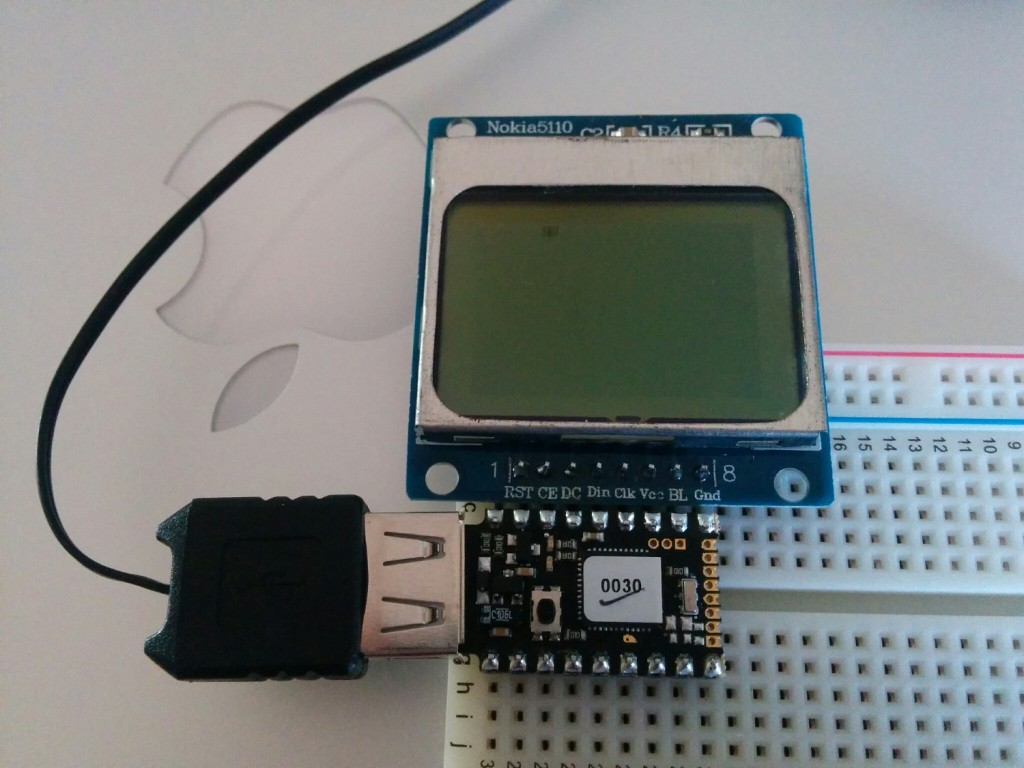# Playing with my espruino pico

About a year ago I had a little fun using an espruino. I recently “kick-started” their more recent product the espruino pico. This one did really well on kickstarter and I got some great “boost” rewards like an LCD screen and some relays. It should be fun to see what this thing can do.Below is a simple program that makes a spot spin around on the LED display that came with the kit. Variables like `A5` or `B10` represent pins on the board, which can be seen in the pico schematic.

This makes use of the PCD8544 driver, which works with the espruino graphics library. This is loosely based on some of the examples on the pico site.

``````A5.write(0); // GND
A7.write(1); // VCC

// http://www.espruino.com/SPI
var spi = new SPI();
spi.setup({ sck:B1, mosi:B10 });

var height = 48; // these are the resolution of the Nokia 5110.
var width = 88;

var rate = 140;
var theta = 0;
var cx = width / 2;
var cy = height / 2 - 1;
var r = cy - 1;
var rx = r * 1.6;
var dTheta = 0.1;

var g = require("PCD8544").connect(spi,B13,B14,B15, function() {
setInterval(function() {
g.clear();
theta += dTheta;
var x = cx + rx * Math.cos(theta);
var y = cy + r * Math.sin(2 * theta);
g.drawLine(x + 1, y, x + 1, y + 1);
g.drawLine(x, y + 1, x + 2, y + 1);
g.flip();
}, rate);
});``````